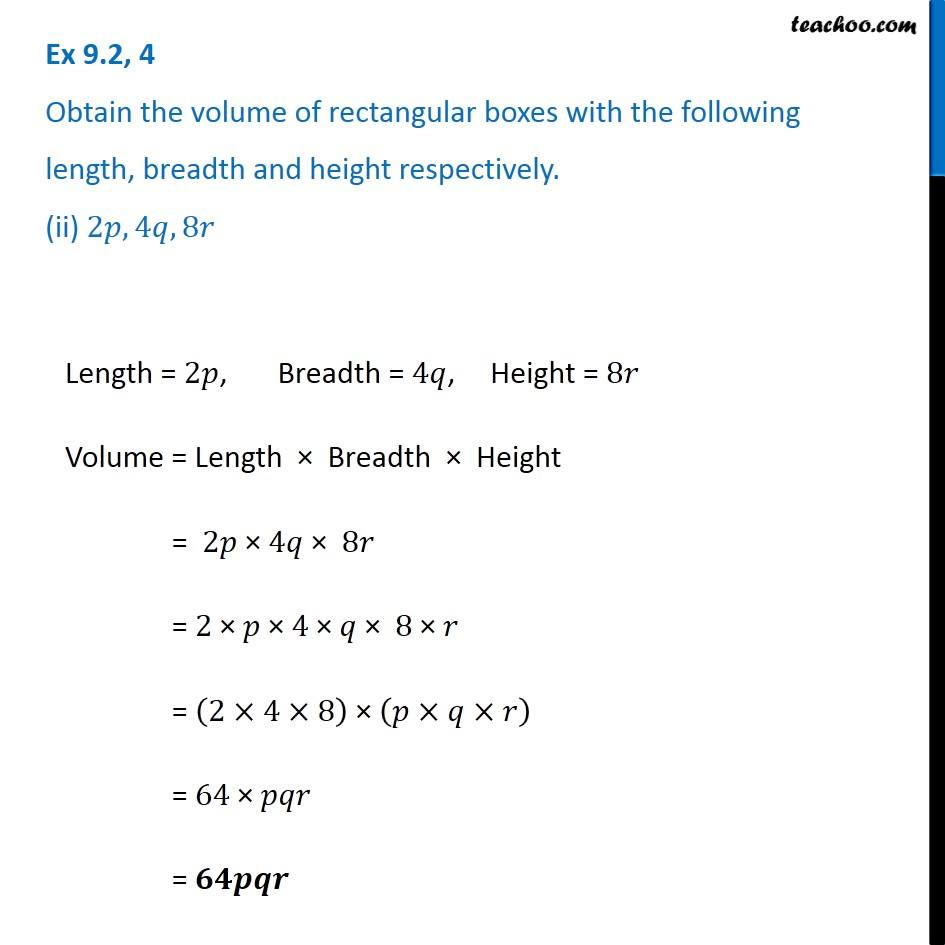Ex 9.2

Chapter 9 Class 8 Algebraic Expressions and Identities
Serial order wiseIntroducing your new favourite teacher - Teachoo Black, at only ₹83 per month

### Transcript

Ex 9.2, 4 Obtain the volume of rectangular boxes with the following length, breadth and height respectively. (ii) 2𝑝, 4𝑞, 8𝑟 Length = 2𝑝, Breadth = 4𝑞, Height = 8𝑟 Volume = Length × Breadth × Height = 2𝑝 × 4𝑞 × 8𝑟 = 2 × 𝑝 × 4 × 𝑞 × 8 × 𝑟 = (2×4×8) × (𝑝×𝑞×𝑟) = 64 × 𝑝𝑞𝑟 = 𝟔𝟒𝒑𝒒𝒓